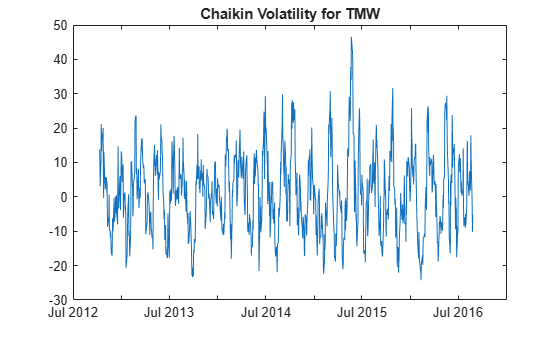# chaikvolat

Chaikin volatility

## Syntax

``volatility = chaikvolat(Data)``
``volatility = chaikvolat(___,Name,Value)``

## Description

example

````volatility = chaikvolat(Data)` calculates the Chaikin volatility from a data series of high and low stock prices.```

example

````volatility = chaikvolat(___,Name,Value)` adds optional name-value pair arguments. ```

## Examples

collapse all

Load the file `SimulatedStock.mat`, which provides a timetable (`TMW`) for financial data for TMW stock.

```load SimulatedStock.mat volatility = chaikvolat(TMW,'NumPeriods',14,'WindowSize',14); plot(volatility.Time,volatility.ChaikinVolatility) title('Chaikin Volatility for TMW')```## Input Arguments

collapse all

Data with high, low, open, close information, specified as a vector, matrix, table, or timetable. For matrix input, `Data` is an `M`-by-`2` matrix of high and low prices stored in the first and second columns. Timetables and tables with `M` rows must contain variables named `'High'` and `'Low'` (case insensitive).

Data Types: `double` | `table` | `timetable`

### Name-Value Arguments

Specify optional pairs of arguments as `Name1=Value1,...,NameN=ValueN`, where `Name` is the argument name and `Value` is the corresponding value. Name-value arguments must appear after other arguments, but the order of the pairs does not matter.

Before R2021a, use commas to separate each name and value, and enclose `Name` in quotes.

Example: ```volatility = chaikvolat(TMW,'NumPeriods',10,'WindowSize',10)```

Period difference, specified as the comma-separated pair consisting of `'NumPeriods'` and a scalar positive integer.

Data Types: `double`

Length of the exponential moving average in periods, specified as the comma-separated pair consisting of `'WindowSize'` and a scalar positive integer.

Data Types: `double`

## Output Arguments

collapse all

Chaikin volatility, returned with the same number of rows (`M`) and the same type (matrix, table, or timetable) as the input `Data`.